# 4. Find the 3 x 3 matrix that produces a rotation by 60° about the point...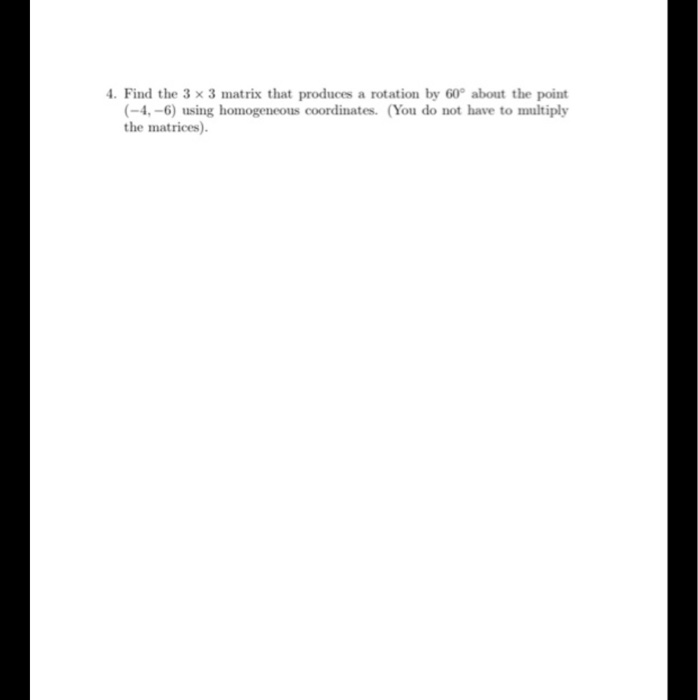4. Find the 3 x 3 matrix that produces a rotation by 60° about the point (-4,-6) using homogeneous coordinates. (You do not have to multiply the matrices).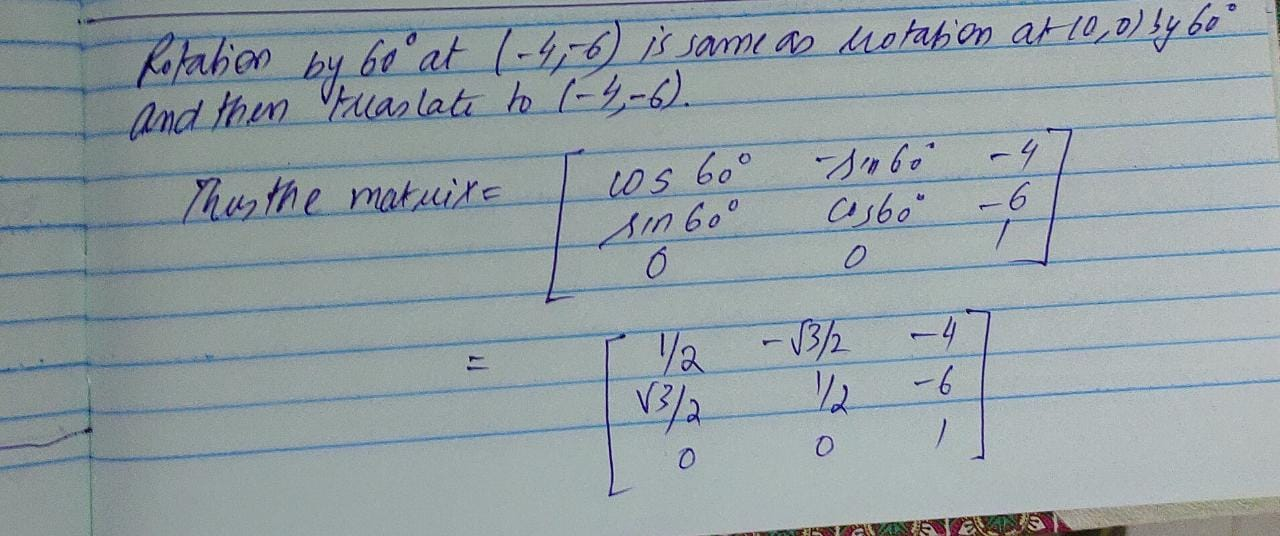#### Earn Coin

Coins can be redeemed for fabulous gifts.

Similar Homework Help Questions
• ### object by 60° about the origin and abount point Aa Find the matrix that represents rotation...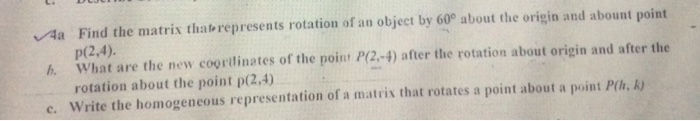object by 60° about the origin and abount point Aa Find the matrix that represents rotation of an P(2,4). What are the new coorilinates of the point P(2,-4) after the rotation about origin and after the rotation about the point p(2,4) Write the homogeneous representation of a matrix that rotates a point about a point P(h, k) h. c. object by 60° about the origin and abount point Aa Find the matrix that represents rotation of an P(2,4). What are...

• ### (4) (a) Determine the standard matrix A for the rotation r of R 3 around the z-axis through the a...(4) (a) Determine the standard matrix A for the rotation r of R 3 around the z-axis through the angle π/3 counterclockwise. Hint: Use the matrix for the rotation around the origin in R 2 for the xy-plane. (b) Consider the rotation s of R 3 around the line spanned by h 1 2 3 i through the angle π/3 counterclockwise. Find a basis of R 3 for which the matrix [s]B,B is equal to A from (a). (c) Give...

• ### 5. Find the matrix (with EXACT values) that produces the rotation of 150° a) About the...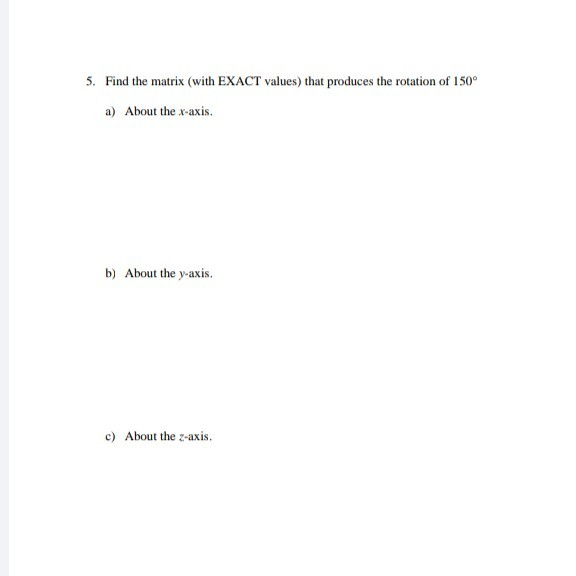5. Find the matrix (with EXACT values) that produces the rotation of 150° a) About the x-axis. b) About the y-axis. c) About the z-axis.

• ### 1 point) Using homogeneous coordinates, the matrix A which rotates the point (-4,-5,-1) about the...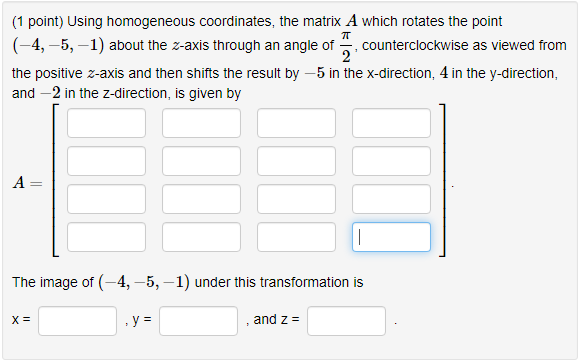1 point) Using homogeneous coordinates, the matrix A which rotates the point (-4,-5,-1) about the z-axis through an angle of counterclockwise as viewed fronm the positive z-axis and then shifts the result by-5 in the x-direction, 4 in the y-direction, and -2 in the z-direction, is given by The image of (-4,-5,-1) under this transformation is 0 , and Z = 1 point) Using homogeneous coordinates, the matrix A which rotates the point (-4,-5,-1) about the z-axis through an angle...

• ### Problem: Given a rotation R of R3 about an arbitrary axis through a given angle find the matrix w...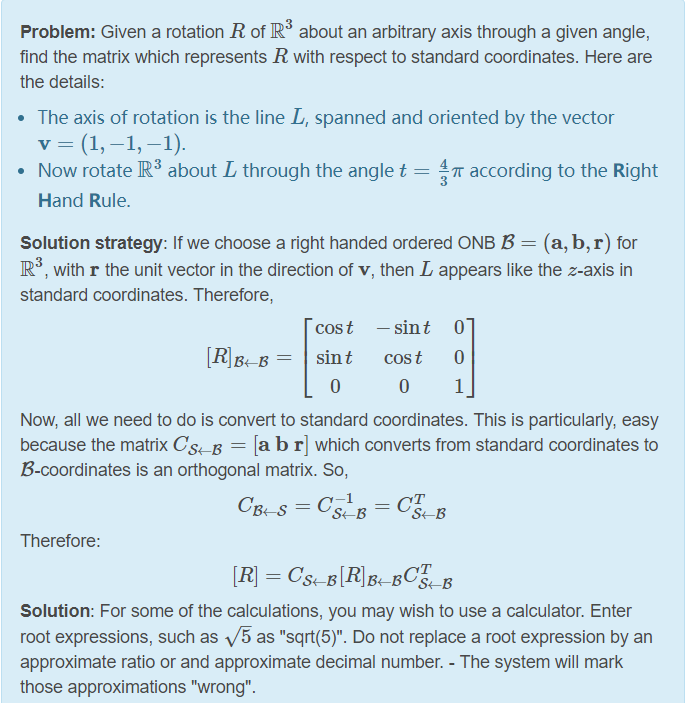Problem: Given a rotation R of R3 about an arbitrary axis through a given angle find the matrix which represents R with respect to standard coordinates. Here are the details: The axis of rotation is the line L, spanned and oriented by the vector v (1,一1,-1) . Now rotate R3 about L through the angle t = 4 π according to the Right 3 Hand Rule Solution strategy: If we choose a right handed ordered ONB B- (a, b,r) for...

• ### Let T : R2 → R2 be the linear transformation given by T(v) = Av that consists of a counterclockwise rotation about the origin through an angle of 30 2, Find the matrix that produces a countercloc...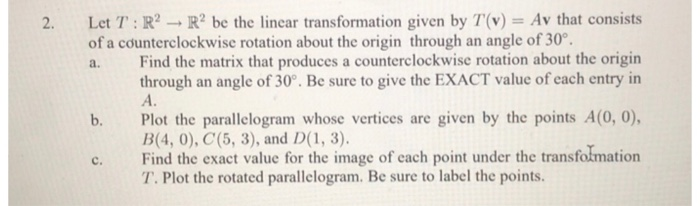Let T : R2 → R2 be the linear transformation given by T(v) = Av that consists of a counterclockwise rotation about the origin through an angle of 30 2, Find the matrix that produces a counterclockwise rotation about the origin through an angle of 30°. Be sure to give the EXACT value of each entry in A. a. b. Plot the parallelogram whose vertices are given by the points A(0, 0), B(4, 0), C(5, 3), and D 1, 3)...

• ### 15.2-1 -- Find an optimal parenthesization of a matrix-chain product whose sequence of dimensions is (5,...

15.2-1 -- Find an optimal parenthesization of a matrix-chain product whose sequence of dimensions is (5, 10, 3, 12, 5, 50, 6). 5. -- Implement Matrix-ChainMultiply(A,s,i,j) using algorithm Matrix-Chain-Order and Matrix-Multiply, where Matrix-Multiply(X,Y, p,q,r) multiplies matrices X and Y, X and Y have pxq and qxr demension, respectively. Given a chain of 6 matrices whose dimensions are given in 15.2-1, and elements are random real numbers from -10 to 10, use Matrix-Chian-ultiply to calculate the product of these matrices.

• ### (1 point) Using homogeneous coordinates, the matrix A which reflects the point (5,-4,-1) across t...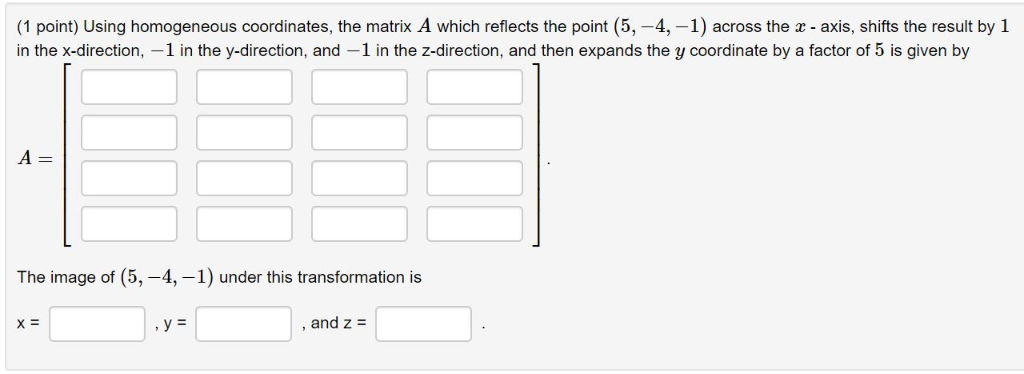(1 point) Using homogeneous coordinates, the matrix A which reflects the point (5,-4,-1) across the x- axis, shifts the result by 1 in the x-direction, -1 in the y-direction, and -1 in the z-direction, and then expands the y coordinate by a factor of 5 is given by A= The image of (5,-4,-1) under this transformation is , and Z = (1 point) Using homogeneous coordinates, the matrix A which reflects the point (5,-4,-1) across the x- axis, shifts the...

• ### (a) Let T: R2 + R2 be counter clockwise rotation by 7/3, i.e. T(x) is the...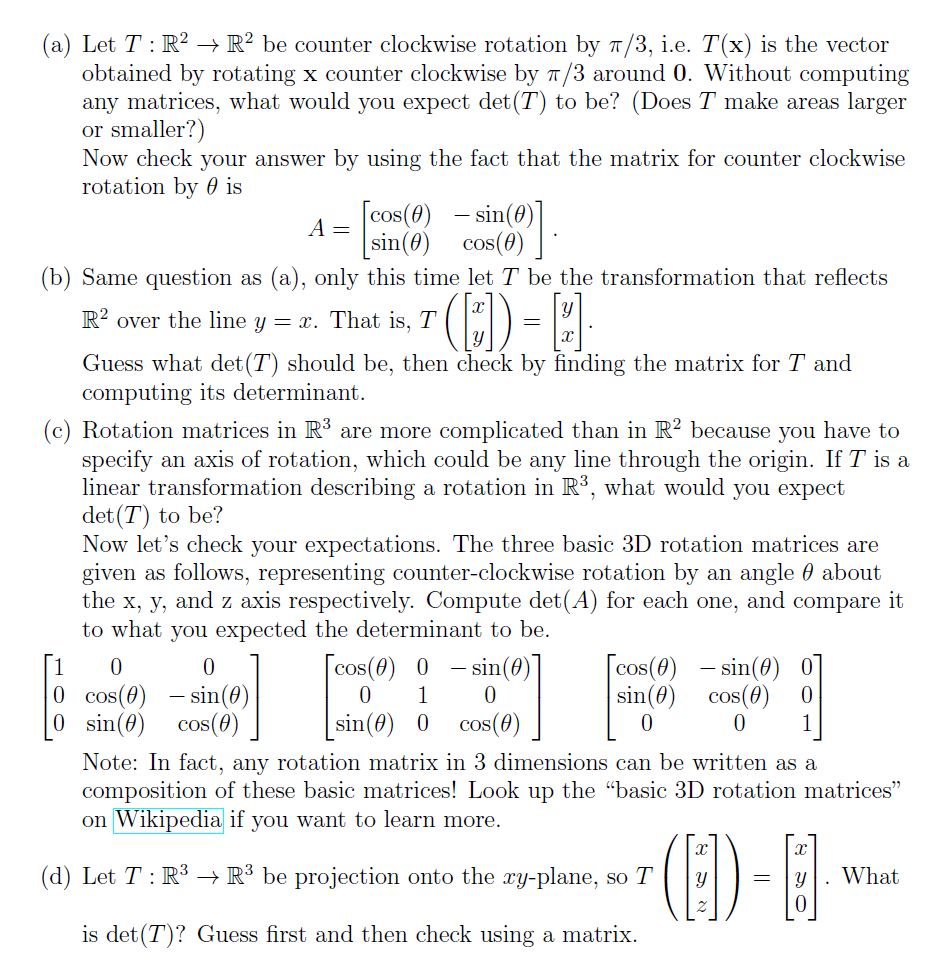(a) Let T: R2 + R2 be counter clockwise rotation by 7/3, i.e. T(x) is the vector obtained by rotating x counter clockwise by 7/3 around 0. Without computing any matrices, what would you expect det (T) to be? (Does T make areas larger or smaller?) Now check your answer by using the fact that the matrix for counter clockwise rotation by is cos(0) - sin(0)] A A= sin(0) cos(0) (b) Same question as (a), only this time let T...

• ### 6a Perform a 60° rotation of point p(3, 5) (a) about the origin and (b) about P(2,2). b. Find the normalization tra...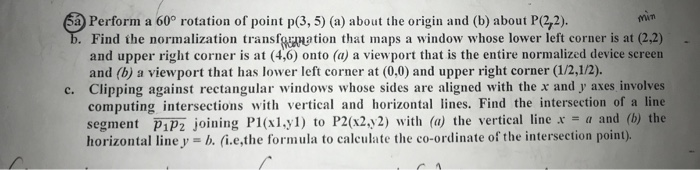6a Perform a 60° rotation of point p(3, 5) (a) about the origin and (b) about P(2,2). b. Find the normalization transfgation that maps a and upper right corner is at (4,6) onto (a) a viewport that is the entire normalized device screen and (b) a viewport that has lower left corner at (0,0) and upper right corner (1/2,1/2). Clipping against rectangular windows whose sides are aligned with the x andy axes involves computing intersections with vertical and horizontal lines....